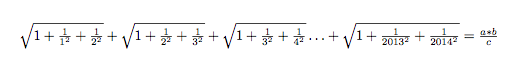# Rooting squares, squaring telescopes, telescoping roots...

Algebra Level 3In the above equation, it is known that a, c, and b are 3 consecutive positive integers with $a < c < b$. What is the value of $a + b - c$ ?

×